# Polarization Volume Gratings

## Introduction

Polarization Volume Gratings (PVGs) have attracted increasing attention in photonics for their beam steering applications. Due to their very high (nearly 100%) diffraction efficiency, large diffraction angle, polarization selectivity, and simple fabrication process, PVGs have found potential in a wide range of novel photonic applications including near-eye displays for AR/VR, optical switching, and laser beam steering. In this article we will describe the simulation of a PVG working at optical wavelengths.

## PVG Overview

Polarization Volume Gratings can be considered as a variety of Volume Bragg Gratings, and are based on patterned cholesteric liquid crystals (CLC). In a conventional CLC, a helical twist is induced along the vertical direction while the LC is homogeneous in the horizontal plane. By contrast, in a PVG another periodic structure is induced in the horizontal plane, as illustrated in Figure 1.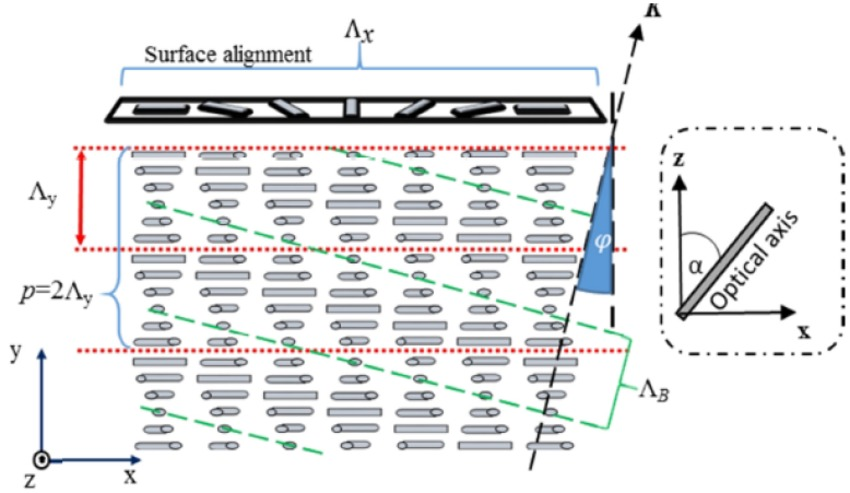Figure 1. Schematic diagram of the PVG . The optical axis rotates in the xz-plane, the rotating angle α changes continuously and periodically along the x&y directions with periods Λx and Λy.

The refractive index distribution of a PVG presents itself as a tilted volume grating with a tilt angle φ. Bragg diffraction can be established when the medium is thick enough to generate sufficient periodical refractive index planes; for this paper, a thickness of 4um, corresponding to ~22 periods, will be used.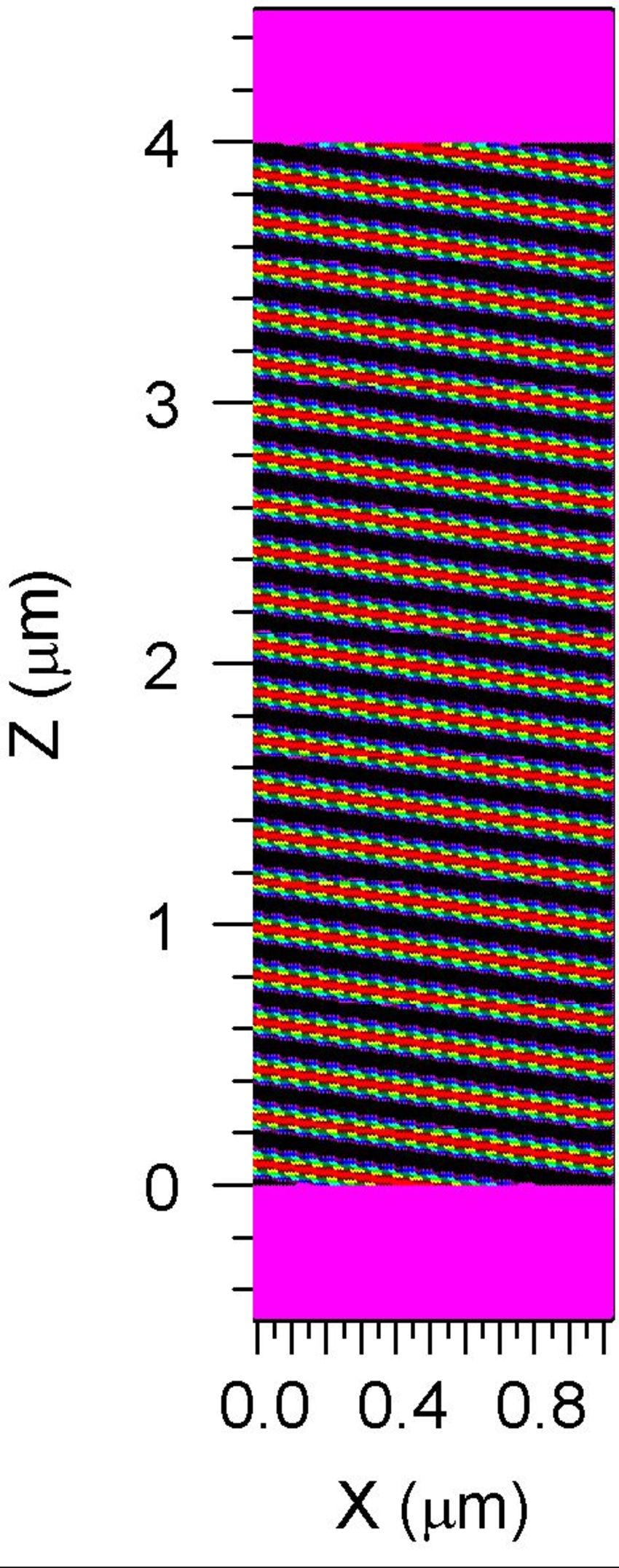Figure 2. PVG real refractive index xx-tensor component profile (Nxx_real).

## PVG Simulation

The above structure is easily created in the RSoft CAD using the RSoft CAD’s crystal axis features. RSoft’s FullWAVE FDTD-based Maxwell equation solver is then used to rigorously simulate the PVG structure. The PVG simulation setup is shown in Figure 3.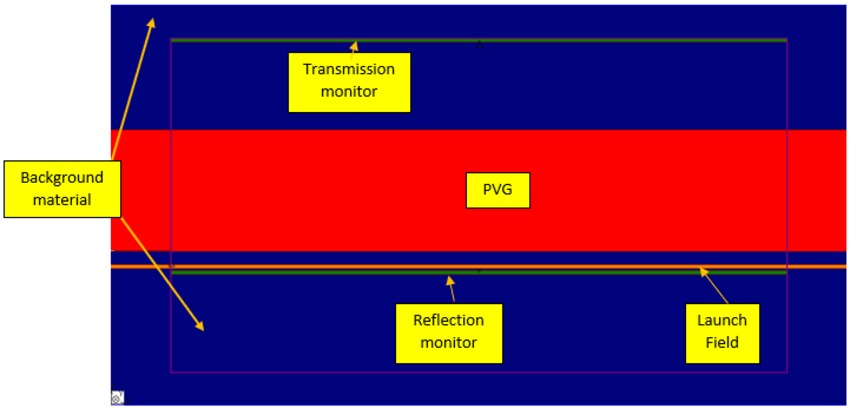Figure 3. PVG simulation setup in the RSoft CAD. The structure consists of a PVG layer in a background medium. A planewave excitation field is launched toward the PVG layer; the transmission & reflection is then measured using field monitors.

## PVG Simulation Results

In this section, we discuss the FDTD simulation results of the PVG structure.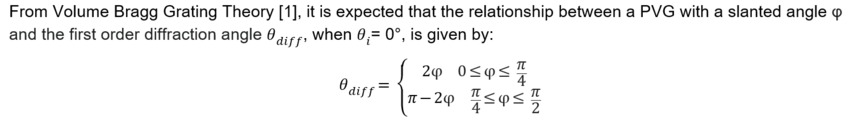From the equation above it is clear that arbitrary diffraction angles can be obtained by adjusting the PVG slant angle φ; i.e, to achieve a desired PVG diffraction angles of (20°, 40°, 60°), a PVG grating tilt angle of (10°, 20°, 30°) should be used. FDTD simulation of the PVG is used to confirm this behavior; the qualitative behavior of the reflected fields can be seen in the FDTD field propagation plots, and FullWAVE’s built-in Diffraction Efficiency vs. Angle feature is used to confirm agreement between simulation & theory for the reflected angle of the 1st diffracted order.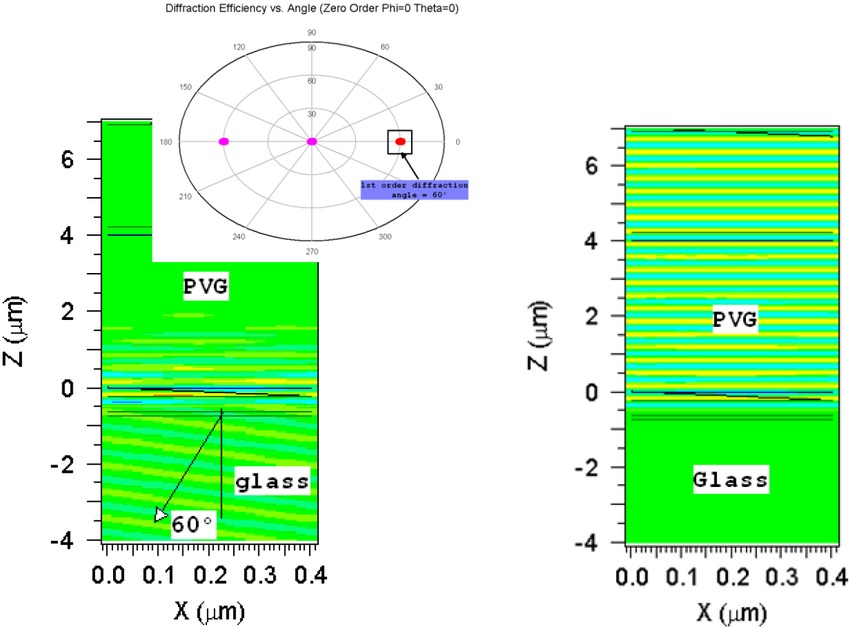Figure 4. Simulated electric field distribution with (left) RHCP incident light, and (b) LHCP incident light. Bragg reflection occurs when the incident beam has same handedness as the twist helix in the reflective PVG (RHC in this simulation). Here we take Δn = 0.2 (ne = 1.7, no = 1.5), PVG thickness d = 4 μm, nglass = 1.57, and operating wavelength λ = 550 nm. The PVG grating itself has a tilt angle of 30°; for this tilt angle, the incident RHCP plane wave is reflected at 60° as measured by FullWAVE’s Diffraction Efficiency vs. Angle feature (left plot inset), this is consistent with expectations from Volume Bragg Grating Theory .

As a major target application of PVGs is for use in near-eye displays for AR/VR applications, it is strongly desired for the PVG to provide uniform beam-steering performance over as wide a range of wavelengths & incident field angles as possible. MOST, RSoft's scanning and optimization utility, is used to investigate the PVG angle & wavelength bandwidth using FDTD as a simulation engine, the results are shown in Figure 5.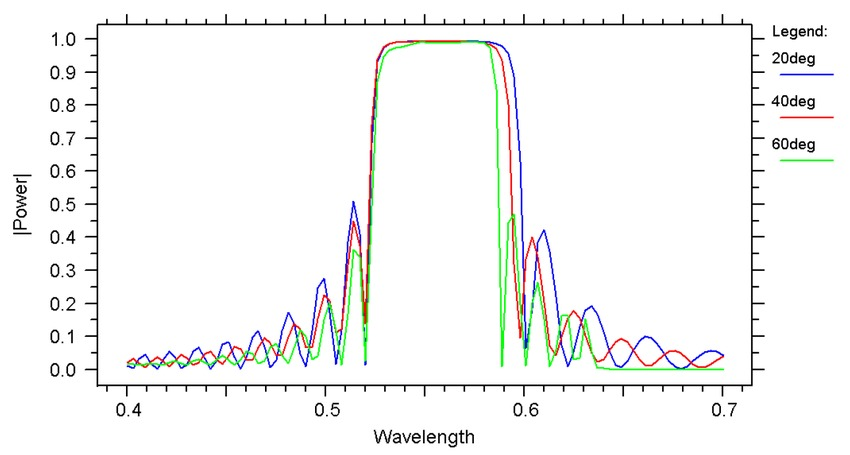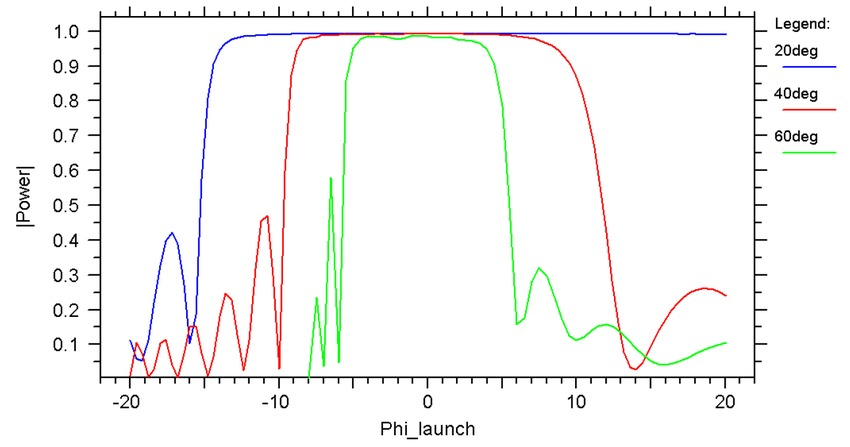Figure 5. (Top, Reflection diffraction efficiency vs. wavelength for a normally incident RHCP planewave) & Figure 6 (Bottom, Reflection diffraction efficiency vs. angle of incidence for a RHCP planewave).

Reflective PVG diffraction behavior for different diffraction angles (20°, 40°, 60°) in glass. The PVG grating tilt angle is set to (10°, 20°, 30°) to achieve the desired diffraction angles of (20°, 40°, 60°). We assume the Bragg wavelength λB = 550 nm at normal incidence, Δn = 0.2 (ne = 1.7, no = 1.5), PVG thickness d = 4 μm, and nglass = 1.57.

As seen in Figure 5, the PVG has nearly 100% diffraction efficiency over a wide wavelength range, and demonstrates performance that is almost independent of the diffraction angles. This ability of the PVG to diffract light to different angles (20°, 40°, 60°) with high diffraction efficiency and constant wavelength bandwidth is a very favorable feature. The diffraction efficiency for different incident angles is depicted in Figure. 6, and shows a wide angular bandwidth that becomes narrower with a larger diffractive angle. This wide angular bandwidth displayed by the PVGs is also a very desirable feature with potential benefits for multiple applications, such as enlarging the field of view for near-eye display applications.